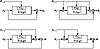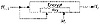Applied Cryptography, Second Edition: Protocols, Algorthms, and Source Code in C (cloth)
(Publisher: John Wiley & Sons, Inc.)
Author(s): Bruce Schneier
ISBN: 0471128457
Publication Date: 01/01/96

Table 18.1
Secure Hash Functions Where the Block Length Equals the Hash Size

Hi = EHi- 1 (Mi) ⊕ Mi
Hi = EHi - 1(MiHi- 1 ) ⊕ MiHi- 1
Hi = EHi- 1(Mi) ⊕ Hi- 1Mi
Hi = EHi- 1(MiHi- 1) ⊕ Mi
Hi = EMi(Hi - 1) ⊕ Hi- 1
Hi = EMi(MiHi - 1 ) ⊕ MiHi - 1
Hi = EMi(Hi - 1 ) ⊕ MiHi - 1
Hi = EMi(MiHi- 1) ⊕ Hi- 1
Hi = EMiHi- 1(Mi) ⊕ Mi
Hi = EMiHi- 1(Hi- 1) ⊕ Hi- 1
Hi = EMiHi- 1(Mi ) ⊕ Hi- 1
Hi = EMiHi- 1(Hi- 1 ) ⊕ Mi

The three different variables can take on one of four possible values, so there are 64 total schemes of this type. Bart Preneel studied them all .

Fifteen are trivially weak because the result does not depend on one of the inputs. Thirty-seven are insecure for more subtle reasons. Table 18.1 lists the 12 secure schemes remaining: The first 4 are secure against all attacks (see Figure 18.9) and the last 8 are secure against all but a fixed-point attack, which is not really worth worrying about.

The first scheme was described in . The third scheme was described in [1555, 1105, 1106] and was proposed as an ISO standard . The fifth scheme was proposed by Carl Meyer, but is commonly called Davies-Meyer in the literature [1606, 1607, 434, 1028]. The tenth scheme was proposed as a hash-function mode for LOKI .

The first, second, third, fourth, ninth, and eleventh schemes have a hash rate of 1; the key length equals the block length. The others have a rate of k/n, where k is the key length. This means that if the key length is shorter than the block length, then the message block can only be the length of the key. It is not recommended that the message block be longer than the key length, even if the encryption algorithm’s key length is longer than the block length.

If the block algorithm has a DES-like complementation property and DES-like weak keys, there is an additional attack that is possible against all 12 schemes. The attack isn’t very dangerous and not really worth worrying about. However, you can solve it by fixing bits 2 and 3 of the key to “01” or “10” [1081, 1107]. Of course, this reduces the length of k from 56 bits to 54 bits (in DES, for example) and decreases the hash rate.

The following schemes, proposed in the literature, have been shown to be insecure.Figure 18.9  The four secure hash functions where the block length equals the hash size.

This scheme  was broken in :

Hi = EMi(Hi - 1)

Davies and Price proposed a variant which cycles the entire message through the algorithm twice [432, 433]. Coppersmith’s attack works on this variant with not much larger computational requirements .

Another scheme [432, 458] was shown insecure in :

Hi = EMiHi- 1 (Hi- 1)

This scheme was shown insecure in  (c is a constant):

Hi = Ec (MiHi - 1) ⊕ MiHi- 1

Modified Davies-Meyer

Lai and Massey modified the Davies-Meyer technique to work with the IDEA cipher [930, 925]. IDEA has a 64-bit block size and 128-bit key size. Their scheme is

Ho = IH, where IH is a random initial value
Hi = EHi- 1,Mi(Hi- 1)

This function hashes the message in blocks of 64 bits and produces a 64-bit hash value (See Figure 18.10).

No known attack on this scheme is easier than brute force.Figure 18.10  Modified Davies-Meyer.

Preneel-Bosselaers-Govaerts-Vandewalle

This hash function, first proposed in , produces a hash value twice the block length of the encryption algorithm: A 64-bit algorithm produces a 128-bit hash.

With a 64-bit block algorithm, the scheme produces two 64-bit hash values, Gi and Hi, which are concatenated to produce the 128-bit hash. With most block algorithms, the block size is 64 bits. Two adjacent message blocks, Li and Ri, each the size of the block length, are hashed together.

G0 = IG, where IG is a random initial value
H0 = IH, where IH is another random initial value
Gi = ELiHi- 1(RiGi- 1 ) ⊕ RiGi- 1Hi- 1
Hi = ELiRi(Hi- 1Gi- 1 ) ⊕ LiGi- 1Hi- 1

Lai demonstrates attacks against this scheme that, in some instances, make the birthday attack trivially solvable [925, 926]. Preneel  and Coppersmith  also have successful attacks against this scheme. Do not use it.

Quisquater-Girault

This scheme, first proposed in , generates a hash that is twice the block length and has a hash rate of 1. It has two hash values, Gi and Hi, and two blocks, Li and Ri, are hashed together.

G0 = IG, where IG is a random initial value
H0 = IH, where IH is another random initial value
Wi = ELi(Gi - 1Ri) ⊕ RiHi- 1
Gi = ERi(WiLi) ⊕ Gi- 1Hi- 1Li
Hi = WiGi- 1

[an error occurred while processing this directive]